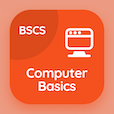Finance Online Courses (BBA)

Cost Accounting Quizzes

Cost Accounting Quiz PDF - Complete

# Estimating Cost Function using Quantitative Analysis Multiple Choice Questions p. 38

Study Estimating Cost Function using Quantitative Analysis multiple choice questions and answers, estimating cost function using quantitative analysis quiz answers PDF 38 to study Cost Accounting course online. Cost Function and Behavior MCQ trivia questions, estimating cost function using quantitative analysis Multiple Choice Questions (MCQ) for online college degrees. "Estimating Cost Function using Quantitative Analysis MCQ" PDF eBook: make versus buy decisions, flexible budget: cost accounting, common costs, payback method, estimating cost function using quantitative analysis test prep for online courses for business management degree.

"For estimation of each cost pool for cost function, the attention must be paid to" MCQ PDF: management hierarchy, cost object hierarchy, price hierarchy, and cost hierarchy for business management degree online. Learn cost function and behavior questions and answers to improve problem solving skills for online BS business administration.

## Estimating Cost Function using Quantitative Analysis Questions and Answers MCQs

MCQ: For estimation of each cost pool for cost function, the attention must be paid to

cost object hierarchy
management hierarchy
price hierarchy
cost hierarchy

MCQ: If the net initial investment is \$6850000 and the uniform increases yearly cash flows is \$2050000, then payback period will be

3.34 years
4.34 years
5.34 years
6.34 years

MCQ: In incremental cost allocation method, the cost object user who is ranked third in ranking order is classified as

First incremental user
primary user
secondary user
second incremental user

MCQ: The subtracted flexible budget amount can form an actual result to calculate

unstated budget variance
flexible budget variance
constant budget variance
static budget variance

MCQ: The difference that exists between total revenues, can be earned from two different alternatives is termed as

independent revenue
incremental revenue
differential revenue
dependent revenue

### More Quizzes from Cost Accounting Course